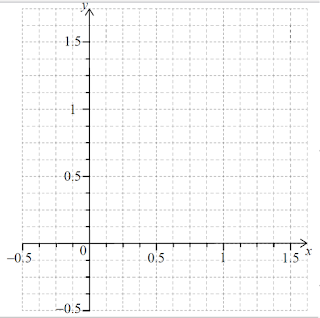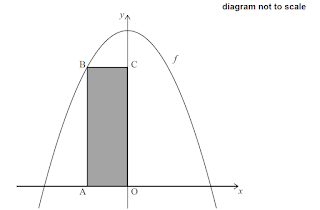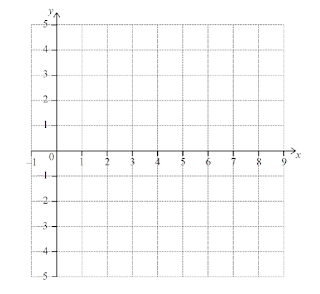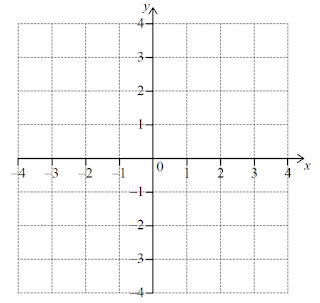# Differetation and its Application (IB SL)

1 (IB/sl/2019/November/Paper1/q5)
[Maximum mark: 6]
Consider the function $f$, with derivative $f^{\prime}(x)=2 x^{2}+5 k x+3 k^{2}+2$ where $x, k \in \mathbb{R}$.
 (a) Show that the discriminant of $f^{\prime}(x)$ is $k^{2}-16$.
 (b) Given that $f$ is an increasing function, find all possible values of $k$. $$

2 (IB/s1/2019/November/Paper1/48)
[Maximum mark: 14]
A small cuboid box has a rectangular base of length $3 x \mathrm{~cm}$ and width $x \mathrm{~cm}$, where $x>0$. The height is $y \mathrm{~cm}$, where $y>0$.
diagram not to scale
The sum of the length, width and height is $12 \mathrm{~cm}$.
 (a) Write down an expression for $y$ in terms of $x$. $$

The volume of the box is $V \mathrm{~cm}^{3}$.
 (b) Find an expression for $V$ in terms of $x$.
 (c) Find $\frac{\mathrm{d} V}{\mathrm{~d} x}$,
 (d) (i) Find the value of $x$ for which $V$ is a maximum. (ii) Justify your answer, 
 (e) Find the maximum volume. 

3 (IB/sl/2019/November/Paper1/q10)
[Maximum mark: 14]
Let $g(x)=p^{r}+q$, for $x, p, q \in \mathbb{R}, p>1$. The point $\mathrm{A}(0, a)$ lies on the graph of $g$. Let $f(x)=g^{-1}(x)$. The point B lies on the graph of $f$ and is the reflection of point A in the line $y=x$,
 (a) Write down the coordinates of $\mathrm{B}$.

The line $L_{1}$ is tangent to the graph of $f$ at $\mathrm{B}$.
 (b) Given that $f^{\prime}(a)=\frac{1}{\ln p}$, find the equation of $L_{1}$ in terms of $x, p$ and $q$.

The line $L_{2}$ is tangent to the graph of $g$ at $\mathrm{A}$ and has equation $y=(\ln p) x+q+1$. The line $L_{2}$ passes through the point $(-2,-2)$. The gradient of the normal to $g$ at $\mathrm{A}$ is $\frac{1}{\ln \left(\frac{1}{3}\right)}$.
 (c) Find the equation of $L_{1}$ in terms of $x$. 

4 $(\mathrm{IB} / \mathrm{s} 1 / 2019 /$ May $/$ paper $1 \mathrm{tz} 2 / \mathrm{q} 9)$
[Maximum mark: 15]
Let $\theta$ be an obtuse angle such that $\sin \theta=\frac{3}{5}$
 (a) Find the value of $\tan \theta$.
 (b) Line $L$ passes through the origin and has a gradient of $\tan \theta$. Find the equation of $L$.

Let $f(x)=\mathrm{e}^{x} \sin x-\frac{3 x}{4}$.
 (c) Find the derivative of $f$.

The following diagram shows the graph of $f$ for $0 \leq x \leq 3$. Line $M$ is a tangent to the graph, of $f$ at point $\mathrm{P}$.
 (d) Given that $M$ is parallel to $L$, find the $x$-coordinate of $\mathrm{P}$. 

5 (IB/s1/2019/May/paper2tz1/q4)
[Maximum mark: 8]
Let $f^{\prime \prime}(x)=(\cos 2 x)(\sin 6 x)$, for $0 \leq x \leq 1 .$
 (a) Sketch the graph of $f^{\prime \prime}$ on the grid below:(b) Find the $x$-coordinates of the points of inflexion of the graph of $f$.
 (c) Hence find the values of $x$ for which the graph of $f$ is concave-down. 

6 (IB/s1/2019/May/paper2tz1/q3)
[Maximum mark: 6]
Consider the function $f(x)=x^{2} \mathrm{e}^{3 x}, x \in \mathbb{R}$.
 (a) Find $f^{\prime}(x)$.
 (b) The graph of $f$ has a horizontal tangent line at $x=0$ and at $x=a$. Find $a$.

7 (IB/s1/2019/May/paper2tz2/q5)
[Maximum mark: 6]
The population of fish in a lake is modelled by the function
$f(t)=\frac{1000}{1+24 \mathrm{e}^{-0.3 t}}, 0 \leq t \leq 30$, where $t$ is measured in months.
 (a) Find the population of fish at $t=10$.
 (b) Find the rate at which the population of fish is increasing at $t=10$.
 (c) Find the value of $t$ for which the population of fish is increasing most rapidly. 

8 (IB/s1/2018/November/Paper1/q10)
[Maximum mark: 16]
Let $f(x)=x^{3}-2 x^{2}+a x+6$. Part of the graph of $f$ is shown in the following diagram.
The graph of $f$ crosses the $y$-axis at the point $\mathrm{P}$. The line $L$ is tangent to the graph of $f$ at $\mathrm{P}$.
 (a) Find the coordinates of $\mathrm{P}$.
 (b) (i) Find $f^{\prime}(x)$.

(ii) Hence, find the equation of $L$ in terms of $a$.
The graph of $f$ has a local minimum at the point $\mathrm{Q}$. The line $L$ passes through $\mathrm{Q}$.
 (c) Find the value of $a$.

9 (IB/s1/2018/November/Paper2/q10)
[Maximum mark: 14]
All lengths in this question are in metres.
Consider the function $f(x)=\sqrt{\frac{4-x^{2}}{8}}$, for $-2 \leq x \leq 2 .$ In the following diagram, the shaded region is enclosed by the graph of $f$ and the $x$-axis.
A container can be modelled by rotating this region by $360^{\circ}$ about the $x$-axis.
 (a) Find the volume of the container.

Water can flow in and out of the container. The volume of water in the container is given by the function $g(t)$, for $0 \leq t \leq 4$, where $t$ is measured in hours and $g(t)$ is measured in $\mathrm{m}^{3}$. The rate of change of the volume of water in the container is given by $g^{\prime}(t)=0.9-2.5 \cos \left(0.4 t^{2}\right)$.
 (b) The volume of water in the container is increasing only when $p < t < q$. (i) Find the value of $p$ and of $q$. (ii) During the interval $p < t < q$, the volume of water in the container increases by $k \mathrm{~m}^{3}$. Find the value of $k$.

When $t=0$, the volume of water in the container is $2.3 \mathrm{~m}^{3}$. It is known that the container is never completely full of water during the 4 hour period.
 (c) Find the minimum volume of empty space in the container during the 4 hour period.

10 $(\mathrm{IB} / \mathrm{s} 1 / 2018 /$ May $/$ paper $1 \mathrm{tz} 1 / \mathrm{q} 8)$
[Maximum mark: 13]
A function $f(x)$ has derivative $f^{\prime}(x)=3 x^{2}+18 x$. The graph of $f$ has an $x$-intercept at $x=-1$.
 (a) Find $f(x)$.
 (b) The graph of $f$ has a point of inflexion at $x=p .$ Find $p$
 (c) Find the values of $x$ for which the graph of $f$ is concave-down. 

11 (IB/s1/2018/May/paper1tz2/q9)
[Maximum mark: 15]
A closed cylindrical can with radius $r$ centimetres and height $h$ centimetres has a volume of $20 \pi \mathrm{cm}^{3}$.
diagram not to scale
 (a) Express $h$ in terms of $r$. 

The material for the base and top of the can costs 10 cents per $\mathrm{cm}^{2}$ and the material for the curved side costs 8 cents per $\mathrm{cm}^{2}$. The total cost of the material, in cents, is $C$.
 (b) Show that $C=20 \pi r^{2}+\frac{320 \pi}{r}$.
 (c) Given that there is a minimum value for $C$, find this minimum value in terms of $\pi$. 

12 (IB/s1/2018/May/paper1tz2/q10)
[Maximum mark: 16]
Consider a function $f$. The line $L_{1}$ with equation $y=3 x+1$ is a tangent to the graph of $f$ when $x=2$.
 (a) (i) Write down $f^{\prime}(2)$. (ii) Find $f(2)$.

Let $g(x)=f\left(x^{2}+1\right)$ and $\mathrm{P}$ be the point on the graph of $g$ where $x=1$.
 (b) Show that the graph of $g$ has a gradient of 6 at $\mathrm{P}$.
 (c) Let $L_{2}$ be the tangent to the graph of $g$ at $\mathrm{P} . L_{1}$ intersects $L_{2}$ at the point $\mathrm{Q}$. Find the $y$-coordinate of $Q$.

13 $(\mathrm{IB} / \mathrm{s} 1 / 2018 /$ May $/$ paper $2 \mathrm{tz} 1 / \mathrm{q} 1)$
[Maximum mark: 5]
Let $f(x)=\ln x-5 x$, for $x>0$
 (a) Find $f^{\prime}(x)$.
 (b) Find $f^{\prime \prime}(x)$. 
 (c) Solve $f^{\prime}(x)=f^{\prime \prime}(x)$. 

 14 (IB/s1/2017/November/Paper1/q6) [Maximum mark: 7] Let $f(x)=15-x^{2}$, for $x \in \mathbb{R}$. The following diagram shows part of the graph of $f$ and the rectangle $\mathrm{OABC}$, where $\mathrm{A}$ is on the negative $x$-axis, $\mathrm{B}$ is on the graph of $f$, and $\mathrm{C}$ is on the $y$-axis.Find the $x$-coordinate of $\mathrm{A}$ that gives the maximum area of $\mathrm{OABC}$.

15 (IB/s1/2017/November/Paper2/q2)
[Maximum mark: 7]
Let $f(x)=\frac{6 x^{2}-4}{\mathrm{e}^{x}}$, for $0 \leq x \leq 7$
 (a) Find the $x$-intercept of the graph of $f$.
 (b) The graph of $f$ has a maximum at the point $\mathrm{A}$. Write down the coordinates of $\mathrm{A}$.
 (c) On the following grid, sketch the graph of $f$.16 $(\mathrm{IB} / \mathrm{sl} / 2017 /$ May $/$ paper $1 \mathrm{tz} 1 / \mathrm{q} 6)$
[Maximum mark: 6]
The following diagram shows the graph of $f^{\prime}$, the derivative of $f$.
The graph of $f^{\prime}$ has a local minimum at $\mathrm{A}$, a local maximum at $\mathrm{B}$ and passes through $(4,-2)$
 (a) The point $\mathrm{P}(4,3)$ lies on the graph of the function, $f$. (i) Write down the gradient of the curve of $f$ at $\mathrm{P}$. (ii) Find the equation of the normal to the curve of $f$ at $\mathrm{P}$.
 (b) Determine the concavity of the graph of $f$ when $4 < x < 5$ and justify your answer. 

17 $(\mathrm{IB} / \mathrm{s} 1 / 2017 /$ May $/$ paper $2 \mathrm{tz} 2 / \mathrm{q} 8)$
[Maximum mark: 15]
Let $f(x)=-0.5 x^{4}+3 x^{2}+2 x$. The following diagram shows part of the graph of $f$.
There are $x$-intercepts at $x=0$ and at $x=p$. There is a maximum at $\mathrm{A}$ where $x=a$, and $\mathrm{a}$, point of inflexion at B where $x=b$.
 (a) Find the value of $p$.
 (b) (i) Write down the coordinates of $\mathrm{A}$. (ii) Write down the rate of change of $f$ at $\mathrm{A}$.
 (c) (i) Find the coordinates of $\mathrm{B}$. (ii) Find the the rate of change of $f$ at $\mathrm{B}$.
 (d) Let $R$ be the region enclosed by the graph of $f$, the $x$-axis, the line $x=b$ and the line $x=a$. The region $R$ is rotated $360^{\circ}$ about the $x$-axis. Find the volume of the solid formed.

18 (IB/s1/2016/November/Paper1/q10)
[Maximum mark: 16]
Let $f(x)=\cos x$.
 (a) (i) Find the first four derivatives of $f(x)$. (ii) Find $f^{(19)}(x)$.

Let $g(x)=x^{k}$, where $k \in \mathbb{Z}$.
 (b) (i) Find the first three derivatives of $g(x)$. (ii) Given that $g^{(19)}(x)=\frac{k !}{(k-p) !}\left(x^{k-19}\right)$, find $p$.

Let $k=21$ and $h(x)=\left(f^{(19)}(x) \times g^{(19)}(x)\right)$.
 (c) (i) Find $h^{\prime}(x)$. (ii) Hence, show that $h^{\prime}(\pi)=\frac{-21 !}{2} \pi^{2}$. $$

19 (IB/s1/2016/November/Paper2/q2)
[Maximum mark: 7]
Let $f(x)=0.225 x^{3}-2.7 x$, for $-3 \leq x \leq 3$. There is a local minimum point at $\mathrm{A}$.
 (a) Find the coordinates of $\mathrm{A}$.
 (b) On the following grid, (i) sketch the graph of $f$, clearly indicating the point $\mathrm{A}$; (ii) sketch the tangent to the graph of $f$ at $\mathrm{A}$.20 (IB/sl/2016/May/paper1tz1/q9)
[Maximum mark: 15]
Let $f^{\prime}(x)=\frac{6-2 x}{6 x-x^{2}}$, for $0 < x < 6$
The graph of $f$ has a maximum point at $\mathrm{P}$.
 (a) Find the $x$-coordinate of $\mathrm{P}$.

The $y$-coordinate of $\mathrm{P}$ is $\ln 27$.
 (b) Find $f(x)$, expressing your answer as a single logarithm.
 (c) The graph of $f$ is transformed by a vertical stretch with scale factor $\frac{1}{\ln 3}$. The image of $\mathrm{P}$ under this transformation has coordinates $(a, b)$. Find the value of $a$ and of $b$, where $a, b \in \mathbb{N}$. $$

21 (IB/s1/2016/May/paper1tz1/q10)
[Maximum mark: 15]
Let $f(x)=\sqrt{4 x+5}$, for $x \geq-1.25$
 (a) Find $f^{\prime}(1)$.

Consider another function $g$. Let $\mathrm{R}$ be a point on the graph of $g$. The $x$-coordinate of $\mathrm{R}$ is 1 . The equation of the tangent to the graph at $\mathrm{R}$ is $y=3 x+6$.
 (b) Write down $g^{\prime}(1)$.
 (c) Find $g(1)$.
 (d) Let $h(x)=f(x) \times g(x)$. Find the equation of the tangent to the graph of $h$ at the point where $x=1$.

22 (IB/sl/2016/May/paper1tz2/q9)
[Maximum mark; 16]
Fred makes an open metal container in the shape of a cuboid, as shown in the following diagram.
The container has height $x \mathrm{~m}$, width $x \mathrm{~m}$ and length $y \mathrm{~m}$. The volume is $36 \mathrm{~m}^{3}$.
Let $A(x)$ be the outside surface area of the container.
 (a) Show that $A(x)=\frac{108}{x}+2 x^{2}$,
 (b) Find $A^{\prime}(x)$.
 (c) Given that the outside surface area is a minimum, find the height of the container.